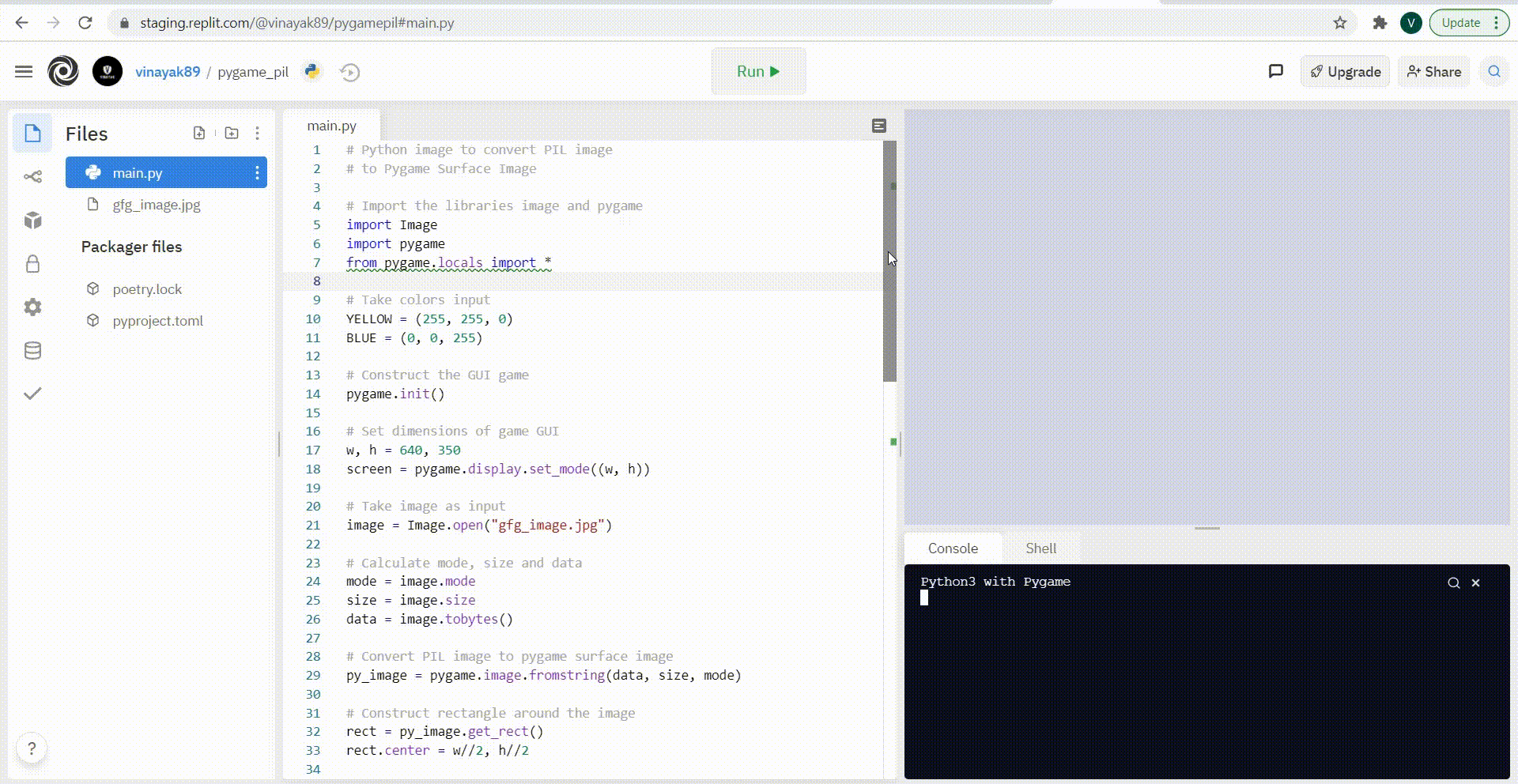# How to Convert PIL Image into pygame surface image?

• Last Updated : 20 May, 2021

In this article, we will know How to Convert PIL Image into pygame surface image.

Pygame Surface Image: A surface that not only has fixed resolution and pixel format but also represents the image in Pygame is known as Pygame Surface Image.

Are you constructing any game in Pygame in which you want to use only Pygame Surface Image as an input? As most of the images entered by the user will be in the PIL Image format, hence you need to convert the PIL images into Pygame surface images before using it. Don’t you know how to convert it into Pygame Surface Image? Don’t worry, it’s too easy to do so. What you need to do is just to use the fromstring() function after taking the PIL image as an input from the user. Read the article given below to know more in detail.

### Approach:

Step 1: First, import the libraries Image and Pygame.

```import Image
import pygame
from pygame.locals import *```

Step 2: Now, take the colors as input that you want to use in the game.

```color_1 = #RGB value of color 1
color_2 = #RGB value of color 2
color_n = #RGB value of color n```

Step 3: Then, construct the GUI game.

`pygame.init()`

Step 4: Further, set the dimensions of your GUI game.

```w, h = #width of game, #height of game
screen = pygame.display.set_mode((w, h))```

Step 5: Next, take the PIL image as input which you wish to convert into Pygame Surface Image.

`image = Image.open("#Enter the image")`

Step 6: Calculate the values of mode which will be used while converting PIL image to pygame surface image.

`mode = image.mode`

Step 7: Further, calculate the value of size which will be used while converting PIL image to pygame surface image.

`size = image.size`

Step 8: Calculate the value of data that will be used while converting PIL image to pygame surface image.

`data = image.tobytes()`

Step 9: Moreover, convert the PIL Image to Pygame Surface Image.

`py_image = pygame.image.fromstring(data, size, mode)`

Step 10: Later on, construct the rectangle around the converted image.

```rect = py_image.get_rect()
rect.center = w//2, h//2```

Step 11: Set the running value for running the game.

`running=True`

Step 12: Set the things which you want your game to do when it is in running state.

```while running:
for event in pygame.event.get():```

Step 12.1: Once the app is in a running state, make it quit if the user wants to quit.

```      if event.type == pygame.QUIT:
running = False```

Step 12.2: Moreover, set the background color which you wish to see in your app.

```   screen.fill(color_1)
screen.blit(py_image, rect)```

Step 12.3: Further, construct the border around the image.

`pygame.draw.rect(screen, color_2, rect, 2)`

Step 12.4: Then, make your app do whatever you want it do while being in a running state.

Step 12.5: After doing everything you wish to do, update the changes done.

`  pygame.display.update()`

Step 13: Finally, quit the GUI game

`pygame.quit()`

## Python

 `# Python image to convert PIL image ` `# to Pygame Surface Image ` ` `  `# Import the libraries image and pygame ` `import` `Image ` `import` `pygame ` `from` `pygame.``locals` `import` `*` ` `  `# Take colors input ` `YELLOW ``=` `(``255``, ``255``, ``0``) ` `BLUE ``=` `(``0``, ``0``, ``255``) ` ` `  `# Construct the GUI game ` `pygame.init() ` ` `  `# Set dimensions of game GUI ` `w, h ``=` `640``, ``350` `screen ``=` `pygame.display.set_mode((w, h)) ` ` `  `# Take image as input ` `image ``=` `Image.``open``(``"gfg_image.jpg"``) ` ` `  `# Calculate mode, size and data ` `mode ``=` `image.mode ` `size ``=` `image.size ` `data ``=` `image.tobytes() ` ` `  `# Convert PIL image to pygame surface image ` `py_image ``=` `pygame.image.fromstring(data, size, mode) ` ` `  `# Construct rectangle around the image ` `rect ``=` `py_image.get_rect() ` `rect.center ``=` `w``/``/``2``, h``/``/``2` ` `  `# Set value of running variable ` `running``=``True` ` `  `# Setting what happens when game is in running state ` `while` `running: ` `    ``for` `event ``in` `pygame.event.get(): ` `       `  `      ``# Close if the user quits the game ` `        ``if` `event.``type` `=``=` `QUIT: ` `            ``running ``=` `False` `     `  `    ``# Set the background color ` `    ``screen.fill(YELLOW) ` `    ``screen.blit(py_image, rect) ` `     `  `    ``# Construct the border to the image ` `    ``pygame.draw.rect(screen, BLUE, rect, ``2``) ` `     `  `    ``# Update the GUI pygame ` `    ``pygame.display.update() ` ` `  `# Quit the GUI game ` `pygame.quit()`

Output:My Personal Notes arrow_drop_up
Recommended Articles
Page :